# OpenCV Series : Target Box Outline Border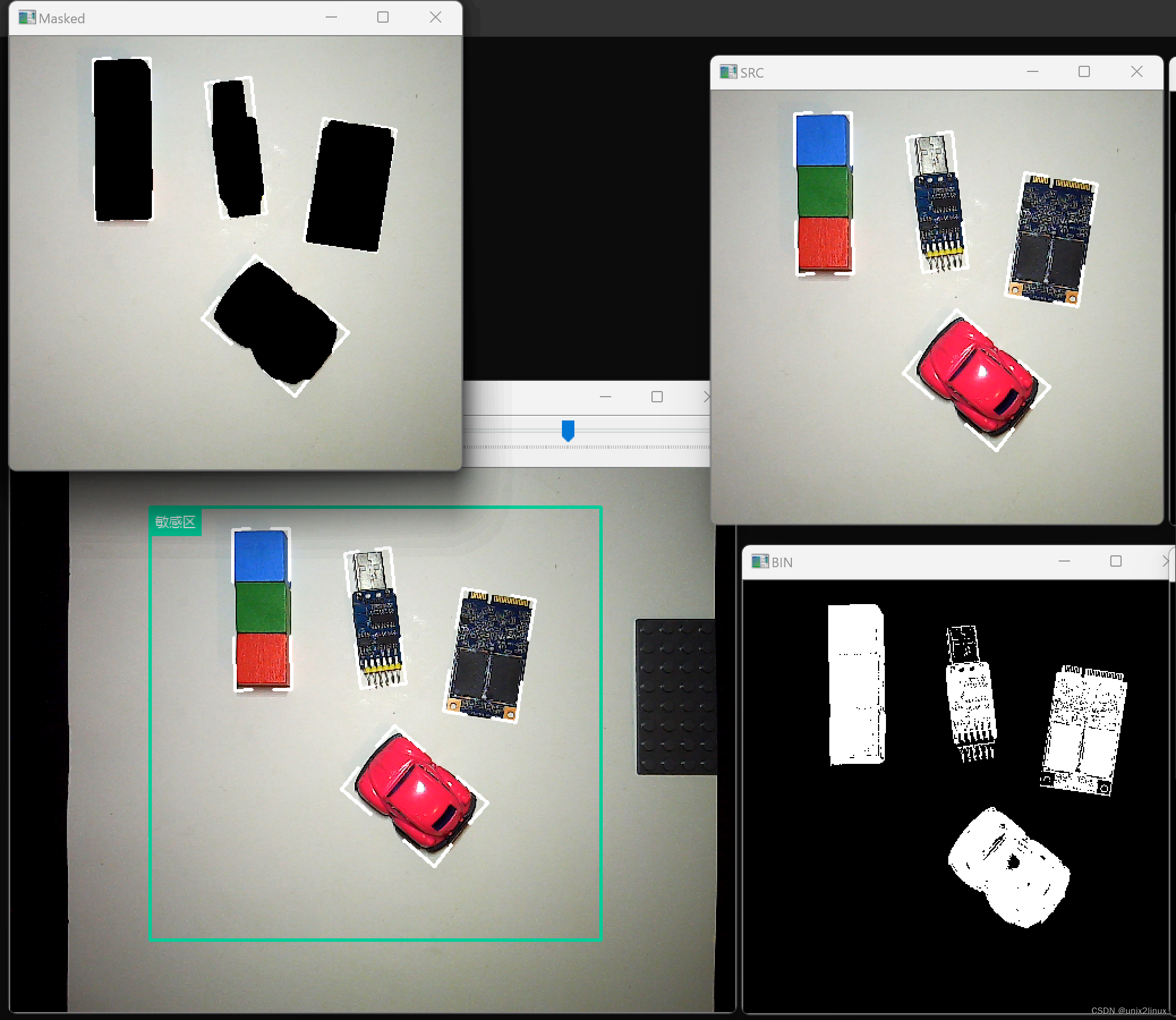``````P1           (255, 000, 000)
P2           (000, 255, 000)
P3           (000, 000, 255)
P4           (000, 000, 000)
``````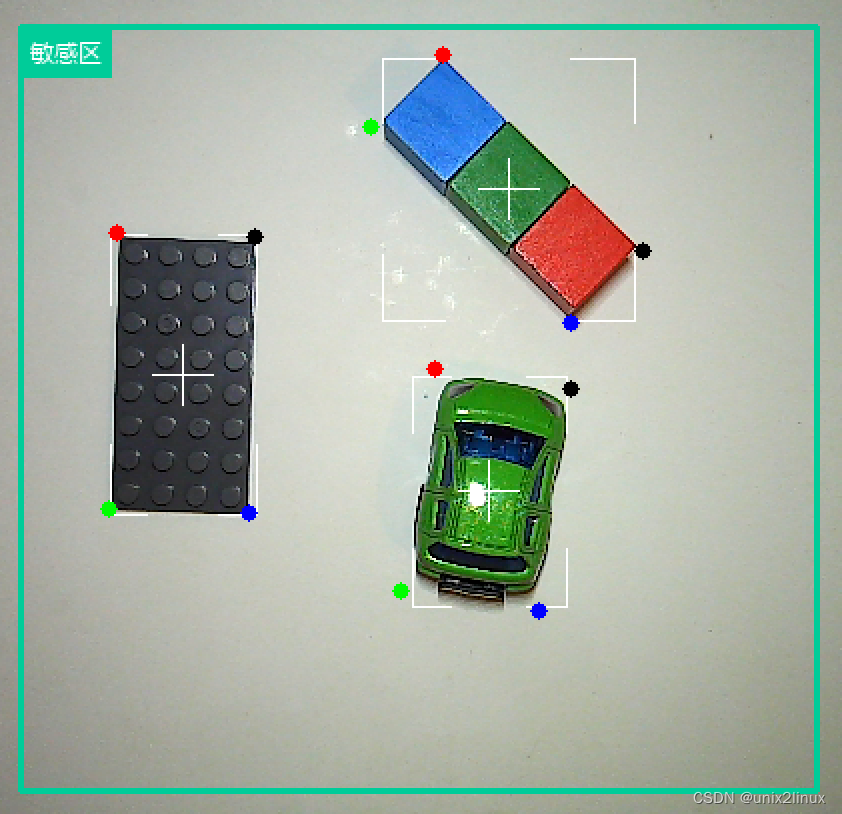``````	rect = cv2.minAreaRect(cnt)
targetColor = roi_color
targetThickness = 1
targetColor = (255, 255, 255)
if lineVerbose:
if True:
cv2.line(photo,  (x, y), (x+w//4, y), targetColor, targetThickness)
cv2.line(photo,  (x, y), (x, y+h//4), targetColor, targetThickness)

cv2.line(photo,  (x+3*w//4, y), (x+w, y), targetColor, targetThickness)
cv2.line(photo,  (x+w, y), (x+w, y+h//4), targetColor, targetThickness)

cv2.line(photo,  (x, y+h), (x+w//4, y+h), targetColor, targetThickness)
cv2.line(photo,  (x, y+h), (x, y+3*h//4), targetColor, targetThickness)

cv2.line(photo,  (x+w, y+h), (x+3*w//4, y+h), targetColor, targetThickness)
cv2.line(photo,  (x+w, y+h), (x+w, y+3*h//4), targetColor, targetThickness)

crossLength = 15
cv2.line(photo,  (x+w//2-crossLength, y+h//2), (x+w//2+crossLength, y+h//2), targetColor, targetThickness)
cv2.line(photo,  (x+w//2, y+h//2-crossLength), (x+w//2, y+h//2+crossLength), targetColor, targetThickness)

``````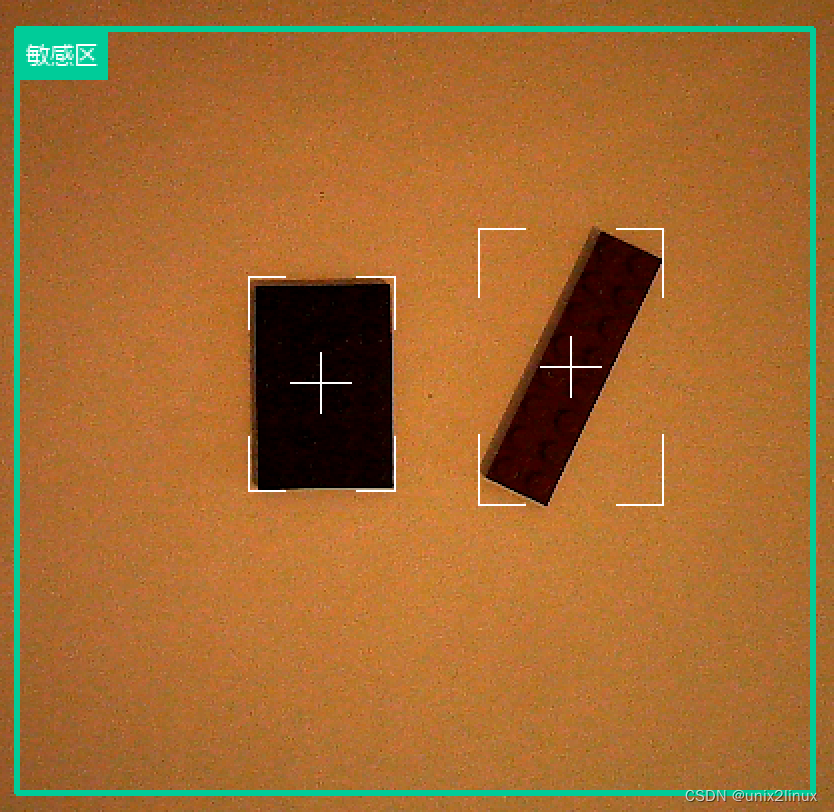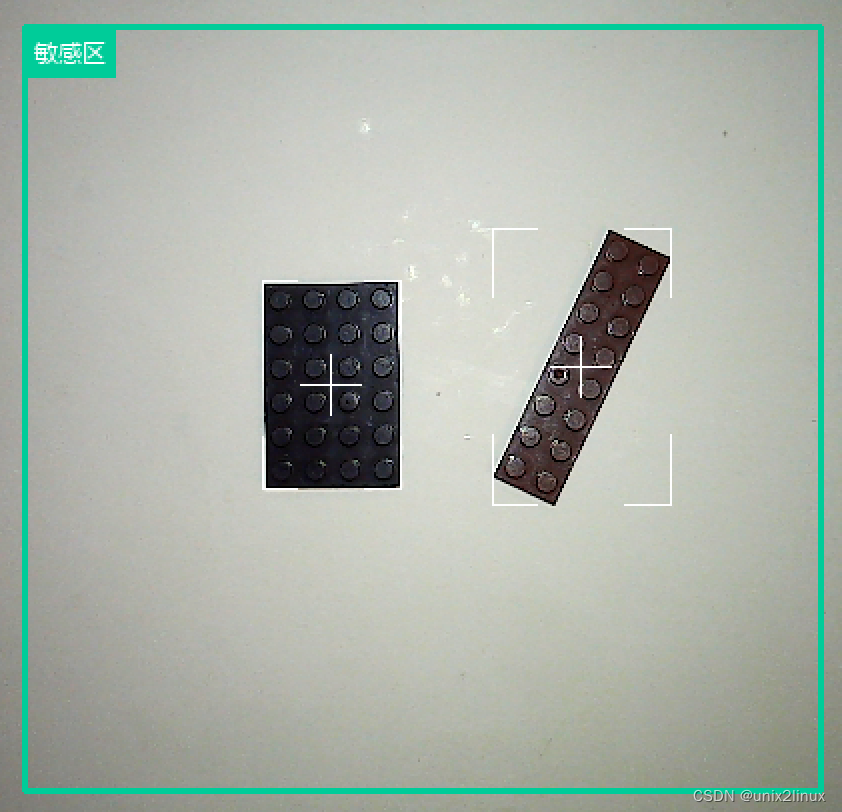``````def lineByAngle(photo, p1, p2, length, color, thickness):
slope = 0 + (p2 - p1) / (p2 - p1)
theta = np.arctan(slope)
degree = int(theta * 57.29577) % 360

length = getDistance(p1, p2) // 4

if (p2 < p1):
pp = (
int(p1 - length * np.cos(theta)),
int(p1 - length * np.sin(theta))
)
else:
pp = (
int(p1 + length * np.cos(theta)),
int(p1 + length * np.sin(theta))
)
cv2.line(photo,  p1, pp, color, thickness)

``````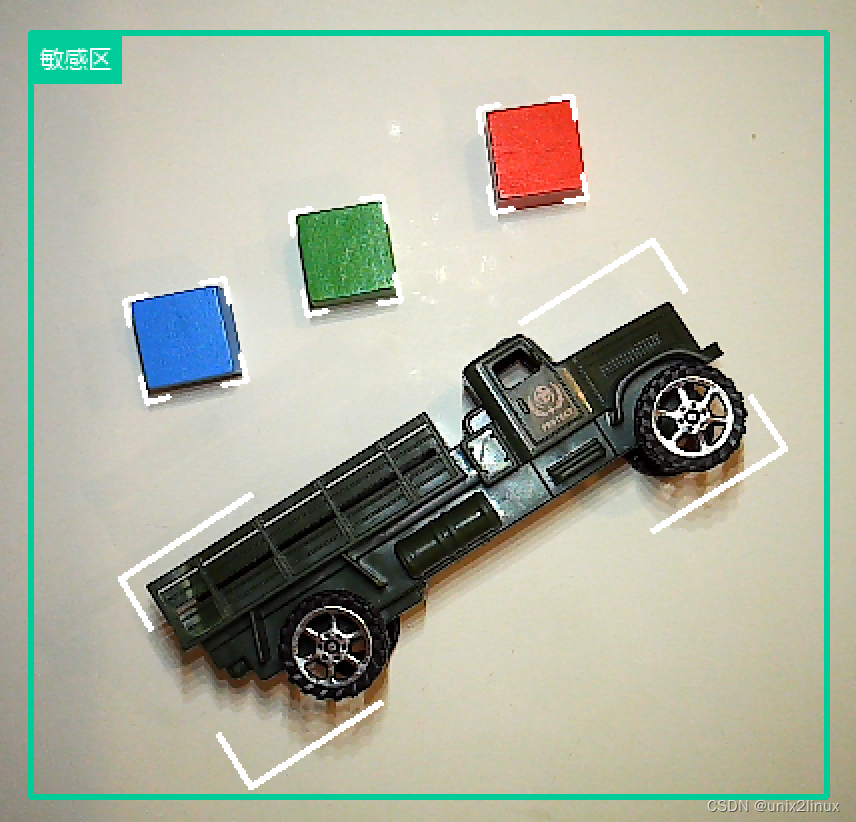### 网站公告